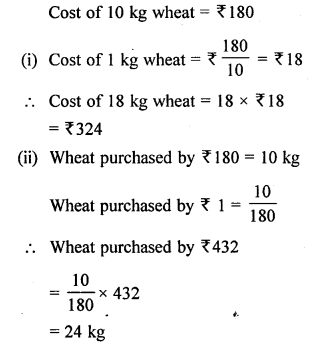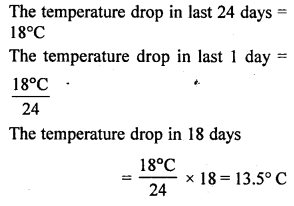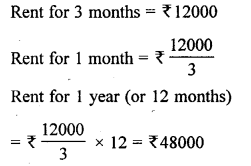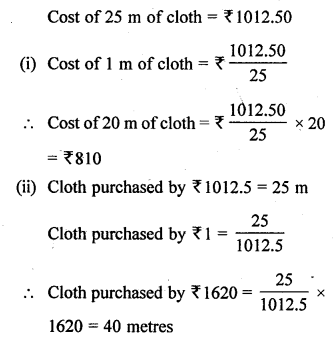## Selina Concise Mathematics Class 6 ICSE Solutions Chapter 13 Unitary Method

Selina Publishers Concise Mathematics Class 6 ICSE Solutions Chapter 13 Unitary Method

APlusTopper.com provides step by step solutions for Selina Concise ICSE Solutions for Class 6 Mathematics. You can download the Selina Concise Mathematics ICSE Solutions for Class 6 with Free PDF download option. Selina Publishers Concise Mathematics for Class 6 ICSE Solutions all questions are solved and explained by expert mathematic teachers as per ICSE board guidelines.

### Unitary Method Exercise 13A – Selina Concise Mathematics Class 6 ICSE Solutions

Question 1.
The price of 25 identical articles is ₹ 1,750. Find the price of :
(i) one article
(ii) 13 articles
Solution:
The price of 25 articles = ₹ 1,750
(i) Price of one article = ₹ $$\frac { 1750 }{ 25 }$$ = ₹ 70
(ii) Now, Price of 13 articles = 13 x ₹ 70 = ₹ 910

Question 2.
A motorbike travels 330 km in 5 litres of petrol. How much distance will it cover in :
(i) one litre of petrol ?
(ii) 2.5 litres of petrol ?
Solution:
(i) Consuming 5 litres petrol in 30 km
Consuming 1 litre petrol, motorbike covers = $$\frac { 330 }{ 5 }$$ km = 66 km
(ii) Consuming 2.5 litres petrol = 66 x 2.5 = 165 km

Question 3.
If the cost of a dozen soaps is ₹ 460.80, what will the cost of:
(i) each soap ?
(ii) 15 soaps ?
(iii) 3 dozen soaps ?
Solution:
(i) Cost of one dozen soap = ₹ 460.80
In one dozen = 12 soaps
Cost of each soap = ₹ $$\frac { 460.80 }{ 12 }$$ = ₹ 38.4
(ii) Cost of 15 soaps = 15 x ₹ 38.4 = ₹ 576
(iii) Cost of 3 dozen soaps = (12 x 3 = 36) = 36 x ₹ 38.4 = ₹ 1382.4

Question 4.
The cost of 35 envelops is ₹ 105. How many envelops can be bought for ₹ 90 ?
Solution:
Envelops purchased by ₹ 105 = 35
Envelopes purchased by ₹ 1 = $$\frac { 35 }{ 105 }$$
In ₹ 90, the envelop will be bought = $$\frac { 35 }{ 105 }$$ x 90 = 30

Question 5.
If the cost of 8 cans of juice is ₹ 280, then what will be the cost of 6 cans of juice ?
Solution:
Cost of 8 cans of juice = ₹ 280
Cost of 1 can of juice = $$\frac { 280 }{ 8 }$$ = ₹ 35
then, cost of 6 cans of juice = 6 x ₹ 35 = ₹ 210

Question 6.
For ₹ 378, 9 cans of juice can be bought, then how many cans of juice can be bought for ₹ 504?
Solution:
In ₹ 378, the juice can bought = 9 cans
In ₹ 504, the cans of juice will be bought = $$\frac { 9 }{ 378 }$$
12 cans of juice can be bought in ₹ 504.

Question 7.
A motorbike travels 425 km in 5 hours. How much distance will be covered by it in 3.2 hours?
Solution:
Distance covered by motorbike = 425 km
Time taken = 5 hours
Distance covered by motorbike in 1 hour = $$\frac { 425 }{ 5 }$$ km/hr = 85 km/hr
Then, distance covered in 3.2 hours = 85 x 3.2 = 272 km/hr

Question 8.
If the cost of a dozen identical articles is ₹ 672, what will be the cost of 18 such articles?
Solution:
Cost of one dozen articles = ₹ 672
Cost of one article = ₹ $$\frac { 672 }{ 12 }$$ = ₹ 56
Cost of 18 articles = ₹ 56 x 18 = ₹ 1008

Question 9.
A car covers a distance of 180 km in 5 hours.
(i) How much distance will the car cover in 3 hours with the same speed ?
(ii) How much time will the car take to cover 54 km with the same speed?
Solution:
Distance covered by car 180 km in 5 hours
(i) Distance covered in 1 hour = $$\frac { 180 }{ 5 }$$ = 36 km
Distance covered in 3 hours = 3 x 36 = 108 km
(ii) To cover a distance of 180 km, time taken = 5 hours
To cover a distance of 1 km, time taken = $$\frac { 5 }{ 180 }$$
To cover a distance of 54 km, time taken = $$\frac { 5 }{ 180}$$ x 54 = 1.5 hours

Question 10.
If it has rained 276 cm in the last 3 days, how many cm of rain will fall in one week (7 days) ?
Assume that the rain continues to fall at the same rate.
Solution:
Rate of rainfall in 3 days = 276 cm
Rainfall in one day = $$\frac { 276 }{ 3 }$$ = 92 cm
Rainfall in one week = 92 x 7 = 644 cm

Question 11.
Cost of 10 kg of wheat is ₹ 180.
(i) What is the cost of 18 kg of wheat ?
(ii) What quantity of wheat can be purchased in ₹ 432 ?
Solution:Question 12.
Rohit buys 10 pens for ₹ 150 and Manoj buys 14 pens for ₹ 168. Who got the pens cheaper?
Solution:
Rohit buys 10 pens = ₹ 150
Cost of one pen = $$\frac { 150 }{ 10 }$$ = ₹ 15
Manoj buys 14 pens = ₹ 168
Cost of one pen = $$\frac { 168 }{ 14 }$$ = ₹ 12

Question 13.
A tree 24 m high casts a shadow of 15 m. At the same time, the length of the shadow casted by some other tree is 6 m. Find the height of the tree.
Solution:
Height of a tree which casts a shadow of 15 m = 24 m
Height of a tree which casts a shadow 24 of 1 m = $$\frac { 24 }{ 15 }$$ m
Height of a tree which casts a shadow of 6 m = $$\frac { 24 }{ 15 }$$ x 6 = 9.6 m

Question 14.
A loaded truck travels 18 km in 25 minutes. If the speed remains the same, how far can it travel in 5 hours?
Solution:
A loaded truck travels in 25 minutes a distance of = 18 km
A loaded truck travels in 1 min a distance of = $$\frac { 18 }{ 25 }$$ km
A loaded truck travels in shows or 300 minutes, a distance of = $$\frac { 18 }{ 25 }$$ x 300 = 216 km

### Unitary Method Exercise 13B – Selina Concise Mathematics Class 6 ICSE Solutions

Question 1.
Weight of 15 books is 6 kg. What is the weight of 45 such books?
Solution:
Weight of 15 books = 6 kg
Weight of 1 book = $$\frac { 6 }{ 15 }$$ kg
Weight of 45 such books = $$\frac { 15 }{ 6 }$$ x 45 = 112.5 kg

Question 2.
A made 84 runs in 6 overs and B made 126 runs in 7 overs. Who made more runs per over?
Solution:
Runs scored by A in 6 overs = 84 runs
Runs scored by A in one over = $$\frac { 84 }{ 6 }$$ = 14 runs
Runs scored by B in 7 overs = 126 runs
Runs scored by B in one over = $$\frac { 126 }{ 7 }$$ = 18 runs
B score more runs per over than A.

Question 3.
Geeta types 108 words in 6 minutes. How many words would she type in half an hour?
Solution:
Words typed by Geeta in 6 minutes = 108
Words typed by Geeta in 1 minute = $$\frac { 108 }{ 6 }$$
Words typed by Geeta in half hour or 30 minutes = $$\frac { 108 }{ 6 }$$ x 30 = 540 words

Question 4.
The temperature dropped 18 degree Celsius in the last 24 days. If the rate of temperature drop remains the same, how many degrees will the temperature drop in the next 18 days?
Solution:Question 5.
Mr. Chopra pays ₹ 12,000 as rent for 3 months. How much does he has to pay for a year if the rent per month remains same?
Solution:Question 6.
A truck requires 108 litres of diesel for covering a distance of 1188 km. How much diesel will be required by the truck to cover a distance of 3300 km?
Solution:Question 7.
If a deposit of ₹ 2,000 earns an interest of ₹ 500 in 3 years, how much interest would a deposit of ₹ 36,000 earn in 3 years with the same rate of simple interest?
Solution:Question 8.
If John walks 250 steps to cover a distance of 200 metres, find the distance covered by him in 350 steps.
Solution:
Distance covered with 250 steps = 200 m
Distance covered with 1 step = $$\frac { 200 }{ 250 }$$ m
Distance covered with 350 steps = $$\frac { 200 }{ 250 }$$ x 350 = 280 m

Question 9.
25 metres of cloth costs ₹ 1,012.50.
(i) What will be the cost of 20 metres of cloth of the same type?
(ii) How many metres of the same kind can be bought for ₹ 1,620?
Solution:Question 10.
In a particular week, a man works for 48 hours and earns ₹ 4,320. But in the next week he worked 6 hours less, how much has he earned in this week?
Solution: